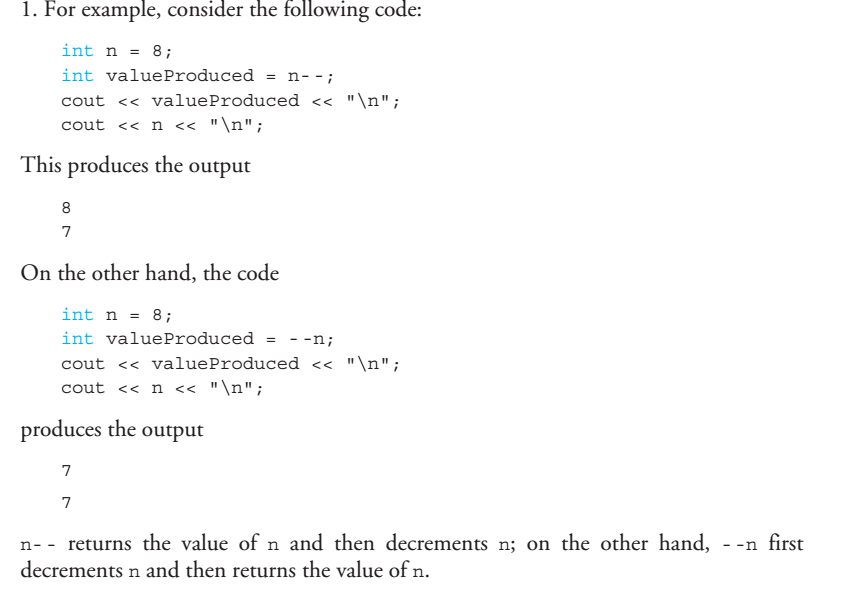### Intro to C++ Part 12

#### How Do I Start a Program?

1.  Identify inputs and outputs.
2. Understand what you asked for ( Ask Questions ).
3. Break the program down. // Functions
4. into steps, writing each step in pseudocode English.
5.  Replace each pseudocode step with C++ codes.

Notes :

1. Most programmers find that placing each name definition on a separate line
is clearer. The word
const is often called a modifier because it modifies (restricts) the
variables being declared.
A variable declared using the
const modifier is often called a declared constant.
• const double RATE = 6.9;
2. static_cast<Type>(Expression)const_cast<Type>(Expression)dynamic_cast<Type>(Expression)reinterpret_cast<Type>(Expression)
3. Notice that the two increment operators in n++ and ++n have the same effect on a
variable
n: They both increase the value of n by 1. But the two expressions evaluate to
different values. Remember, if the
++ is before the variable, the incrementing is donebefore the value is returned; if the ++ is after the variable, the incrementing is done afterthe value is returned.
•4. You cannot apply the increment and decrement operators to anything other than a
single variable. Expressions such as
(x + y)++, --(x + y), 5++, and so forth, are all
illegal in C++.